## A single force acts on a 4.0 kg particle-like object in such a way that the position of the object as a function of time is given by x = 3.0

Question

A single force acts on a 4.0 kg particle-like object in such a way that the position of the object as a function of time is given by x = 3.0t − 4.0t 2 + 1.0t 3, with x in meters and t in seconds. Find the work done on the object by the force from t = 0 to t = 8.0 s.

in progress 0
1 month 2021-08-06T05:48:59+00:00 2 Answers 0 views 0

31.232 kJ

Explanation:

Given,

mass of the particle, m = 4 Kg

Position of object as the function of time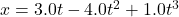Work done by the object by the force from t = 0 to t = 8.0 s =?

we know,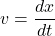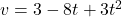velocity at t = 0 s

u = 3 – 8 x 0 + 3 x 0 = 3 m/s

velocity at t= 8 s

v = 3 – 8 x 8 + 3 x 8 x 8

v = 125 m/s

Work done is equal to change in KE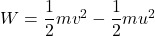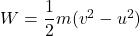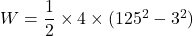W = 31.232 k J

Hence, work done is equal to 31.232 kJ

34304 Joule

Explanation:

mass of particle, m = 4 kg

x = 3t – 4t² + t³

Let v is the velocity

v = dx/dt = 3 – 8t + 3t²

Let a is the acceleration

a = dv/dt = – 8 + 6t

Work is defined as the product of force.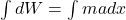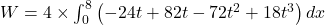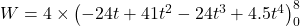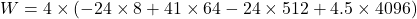W = 4 x (- 192 + 2624 – 12288 + 18432)

W = 34304 Joule